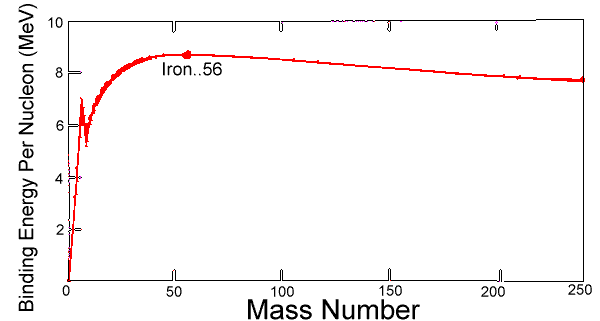# Terms Associated with Nucleus

Atomic mass unit (amu): The mass of nucleus is measured in the unit of amu. One amu is defined asth of mass os c12 isotope which is equal to 1.6×10-27 kg.

Energy released by the mass defect of 1 amu = mc2

= 1.66×10-27 × (3×108)2

= 931×106 ev

= 931 Mev

Mass defect (ΔM): When nucleons combine to form a nucleus, mass is not conserved. The total mass of constituent particles of the nucleus is greater than the combined mass. This difference of mass is known as mass defect. Let ZXA be a nucleus having combined mass M. Then mass defect m is given by:

ΔM =  Zmp + (A – Z)mn – M

Binding energy: Nucleus consists of protons and neutrons within a very small space. There is a force of repulsion between the protons. Despite of this they are forced to be accumulated at the nucleus. Large amount of energy is required to bind the nucleus which is known as binding energy.

This binding energy arises from mass defect i.e. it is the energy obtained by the loss of mass. If m be the mass defect then,

Binding Energy = Δmc2

Or, Binding Energy = [Zmp + (A – Z)mn – M] c2

Binding energy per nucleon: The binding energy available per nucleon is known as binding energy per nucleon(BE/A).  It determines the stability of a nucleus. The greater the value of binding energy per nucleon, the higher will be the stability of nucleus.

The variation of binding energy per nucleus with atomic mass is shown in the graph below.Graph showing variation of energy per nucleon with mass number

As the atomic mass increases, binding energy per nucleon attains a maximum value for iron (26Fe56) having value of 8.79 meV. This value slowly decreases and is minimum for Uranium 92U238 for a value of 7.6meV. This shows that iron is the most stable element and uranium is the most unstable element.

The unstable elements such as uranium slowly decay emitting radiation from nucleus. Such element is called radioactive elements.

Do you like this article ? If yes then like otherwise dislike :